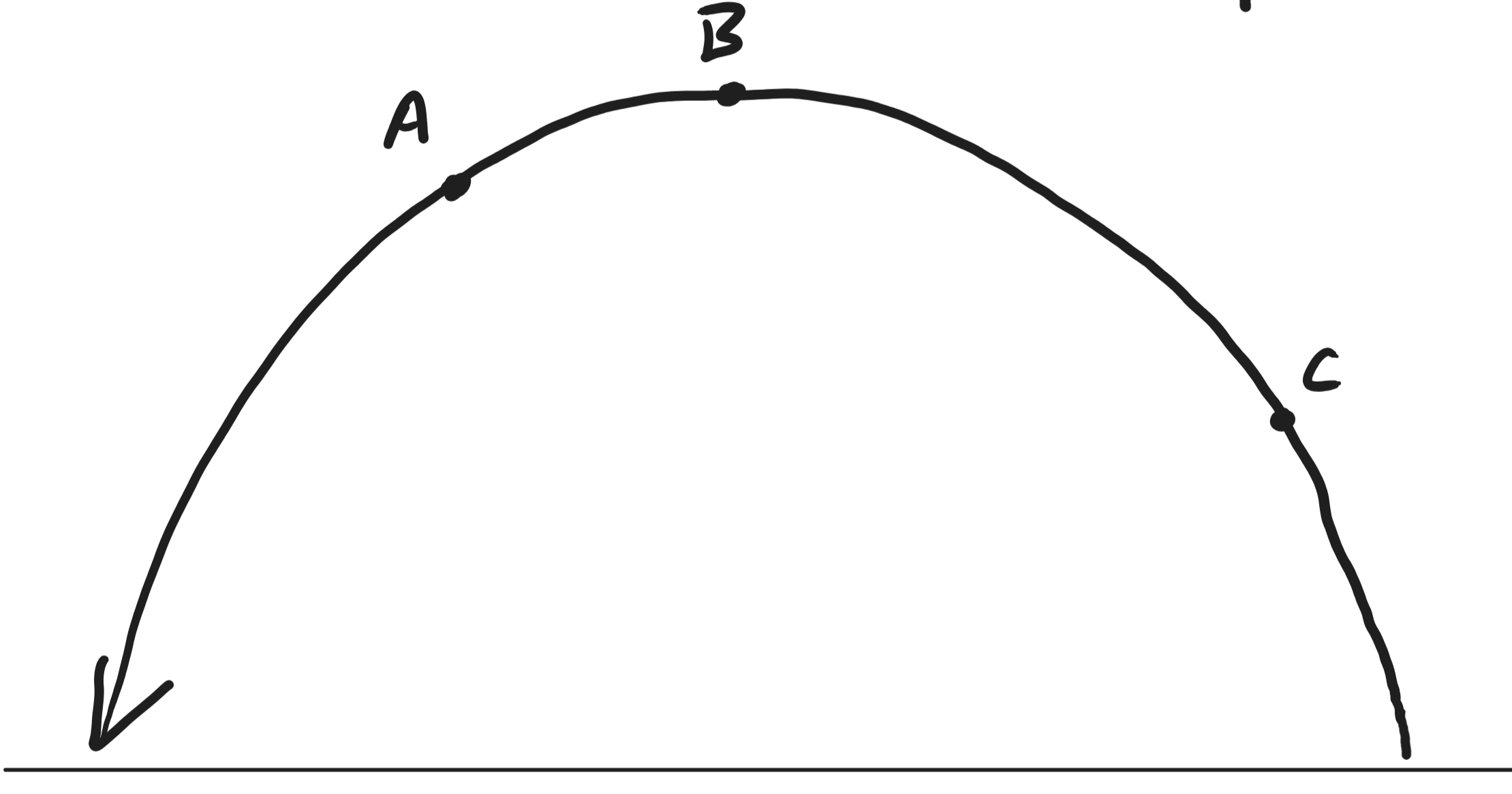﻿ Untitled 1

1. 1m/s = _____ mph

Identify the component and resultant vectors.  Draw a head-to-tail diagram for each one.

1. A helicopter's pilot steers the helicopter on a heading 20 degrees east of North with an air speed (speed in still air) of 150 knots.  The helicopter is traveling westward at a rate of 162 knots.  What is the velocity of the air around the helicopter?

2. An explorer practicing orienteering on the deck of a ship has a velocity of 2.5m/s in a direction 28 degrees North of East.  The explorer is following a compass heading to the Northwest, and if the ship were still, the explorer's speed would be 3m/s.  What is the ship's velocity?

3. At its southern edge, a spinning merry-go-round has a velocity of 10m/s eastward.  A child standing at this position heads toward the center of the merry-go-round.  The child's speed on a still merry-go-round is 4m/s.  What is the child's velocity?1. What is the minimum amount of information that you would need to be provided in order to calculate the x and y velocities of a projectile at all points A, B, and C?  How many individual numbers would you need to be provided?
2. What kinds of problems could we make from a diagram like this?
1. Symmetric?
2. Asymmetric?
3. Half symmetric?